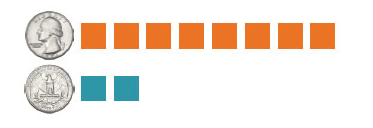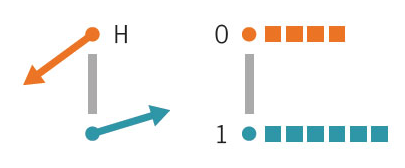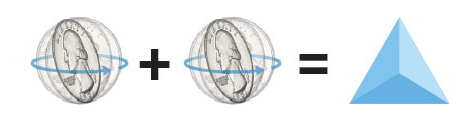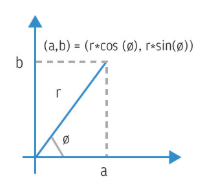When approaching quantum computing from a computer science perspective, it may seem intuitive to begin by comparing quantum computers directly with their classical counterpart. However, many who attempt to learn this way (myself included) end up more confused than informed, especially after encountering the complex mathematical and physics notation present in popular literature. Instead, we will assume a basic understanding of how classical computers function, and discuss the unique qualities of a quantum computation separately. Although this distinction is a subtle, a quantum-focused approach is arguably more enlightening than a direct comparison.

It is worth noting that this is a difficult subject, and thus we encourage beginners to surround themselves with a healthy mix of both literature and examples (see the Activity Corner for additional resources). Understanding quantum state takes time and effort, and it's normal to be confused when starting out. As Richard Feynman said:

"Nature isn't classical . . . and if you want to make a simulation of Nature, you'd better make it quantum mechanical, and by golly it's a wonderful problem, because it doesn't look so easy."

## Quantum State

Let's start by looking at the process of tossing a fair coin. We can regard this process as random, with two possible outcomes: heads or tails. When one outcome of the random process has a greater probability of being measured, we call the coin biased. The bias is an unknown factor which can skew the probability from what we would expect from a fair coin. The bias cannot be determined with only a single coin flip, so the coin would need to be flipped multiple times to get a more precise estimation of what the bias is. The frequency of the outcomes from the coin flips will provide information as a set of probabilities, which must add up to 1.We can think of a biased coin as a probabilistic bit — i.e. there is some randomness to the result, as opposed to deterministically measuring 0 and 1. The probabilities associated with each outcome can be thought of as the parameters of a random process before sampling it, similar to a spinning coin before landing on one of its sides. When the number of possible outcomes is greater than one, we refer to the state as being in superposition of those outcomes. While this term is often used in quantum computing, this idea is not unique and exists for any probabilistic system.

A quantum bit (abbreviated qubit or qbit) is a generalized version of the probabilistic bit. Instead of associating probabilities with each outcome (as we do in the probabilistic bit), we associate 2-dimensional vectors or arrows, which are called amplitudes. The probabilities of the outcomes are correlated to the magnitudes of their corresponding amplitudes. More precisely, the probability of an outcome is the square of the length of its corresponding arrow. Therefore, we can use arrows to represent each outcome of a qubit.

A qubit starts in the default state, where the probability of measuring 0 is 1 (e.g. the coin always turns up heads). The default state is one of the basis states, which correlate directly with a possible outcome of the quantum state. There are two basis states for a single qubit: 0 and 1 (in quantum mechanics these are denoted |0> and |1>, but there is no benefit to making this distinction from a computing perspective). We define a quantum state by its basis states and corresponding amplitudes, much like a dictionary of key-value pairs. The arrow notation discussed above can therefore be spoken of interchangeably with the quantum state itself.

With a classical system we can observe its current state at any time without affecting it. But the state of a quantum system randomly collapses to one of its possible outcomes when measured. We can never observe the quantum state directly — measurement returns a binary string, and the state after observation is the basis state that corresponds to that binary string (the state before measuring is destroyed). In order to retrieve any meaning from the state of a quantum system, its state has to be recreated and measured multiple times, and the random results have to be interpreted as a pattern in the context of the given problem (e.g. flipping a coin over and over again to determine its bias).## Quantum Systems

A quantum computing system is comprised of multiple qubits and has an associated state. The state consists of one amplitude for each possible measurement outcome, and for a quantum system with n qubits, there are 2n outcomes. The simplest system contains only a single qubit, and is the building block with which larger systems are composed. It is crucial that one does not think of a quantum system in terms of the state of its individual qubits, but instead to consider the state as a whole. If we continue the example using a coin, we can think of the composition of the quantum system as quantum coins combining into a die.We don't discuss the outcomes of each individual coin (or qubit) separately. Each face corresponds to a possible combination of heads or tails and has its own probability of being measured. When all outcomes are equally likely to be measured (like in this example), we define the state as being in equal superposition. While equal superposition has its uses (e.g. sampling), typically we want to eliminate some of the possible outcomes, the details of which are problem-specific.

Using the example of a quantum die, let's assume we only want the die to return one of two results: 00 or 11. In a quantum system this can be done with entanglement. Specifically, we force one qubit's measurement to match the other, eliminating the outcomes 01 and 10. The result is effectively a fair coin — there is a 50/50 chance of measuring 00 or 11, which can be classically post-processed into heads (0) or tails (1).

Working with a quantum system is similar to playing a slot machine. We have a desired outcome (or event), a series of operations we are allowed to perform on the qubits and a limited number of available qubits to work with. The challenge is to construct a quantum state that gives the desired answer in the least amount of measurements, which manifests as a balance between accuracy and computation time.

## Superposition

A state in superposition is like a spinning coin or a cast die. Because the coin has yet to stop spinning (or the die has yet to land on a face), more than one outcome is possible. This is sometimes called a general superposition. States can also be in equal superposition (all outcomes are equally likely - the coin is fair, the die is unbiased, etc.).

If we have a spinning, biased coin, we have a general superposition (there are two possible outcomes, but one is more likely than another). If we have a spinning, fair coin, we have an equal superposition (all outcomes are possible, and they are equally likely to be measured). Note that an equal superposition is just a general superposition with an additional equality constraint.

## Complex Numbers

A complex number is comprised of a real part a and an imaginary part b, and can be written in the form a+b∗i. It can also be written in polar form r∗(cos(ϴ)+ i∗sin(ϴ)), where r is the length of the arrow and ϴ is the angle between the arrow and the x-axis, also called the phase of the complex number.

The amplitudes of a quantum state are complex numbers, thus the association with 2-dimensional arrows.